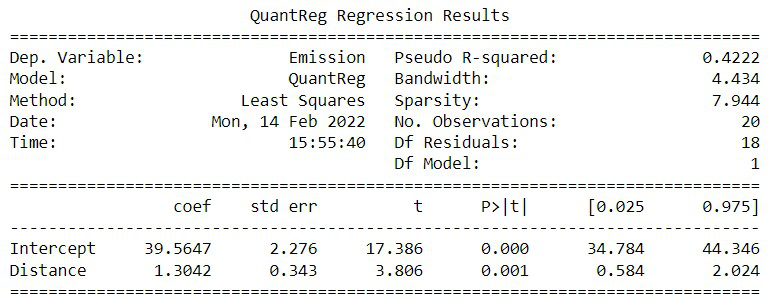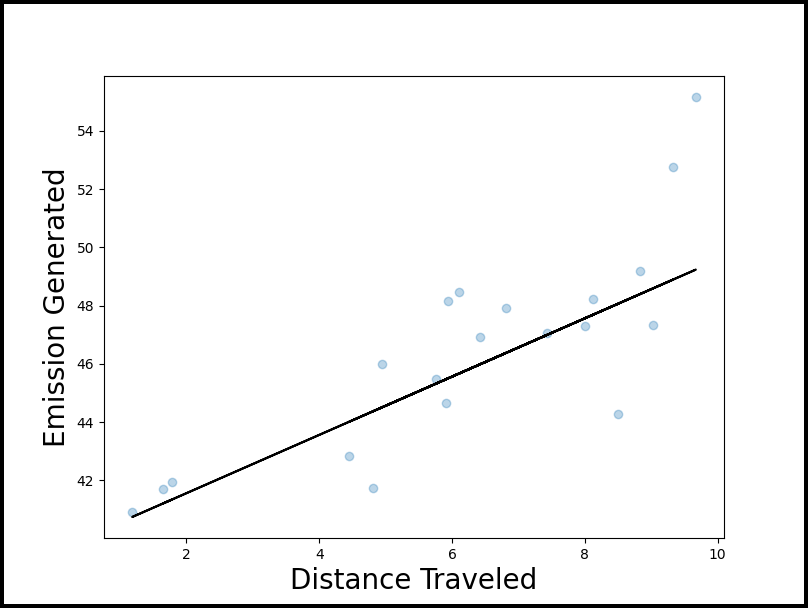# How to Perform Quantile Regression in Python

In this article, we are going to see how to perform quantile regression in Python.

Linear regression is defined as the statistical method that constructs a relationship between a dependent variable and an independent variable as per the given set of variables. While performing linear regression we are curious about computing the mean value of the response variable. Instead, we can use a mechanism known as quantile regression in order to compute or estimate the quantile (percentile) value of the response value. For example, 30th percentile, 50th percentile, etc.

## Quantile regression

Quantile regression is simply an extended version of linear regression. Quantile regression constructs a relationship between a group of variables (also known as independent variables) and quantiles (also known as percentiles) dependent variables.

## Perform quantile regression in Python

Calculation quantile regression is a step-by-step process. All the steps are discussed in detail below:

### Creating a dataset for demonstration

Let us create a dataset now. As an example, we are creating a dataset that contains the information of the total distance traveled and total emission generated by 20 cars of different brands.

## Python3

 `# Python program to create a dataset`   `# Importing libraries` `import` `numpy as np` `import` `pandas as pd` `import` `statsmodels.api as sm` `import` `statsmodels.formula.api as smf` `import` `matplotlib.pyplot as plt`   `np.random.seed(``0``)`   `# Specifying the number of rows` `rows ``=` `20`   `# Constructing Distance column` `Distance ``=` `np.random.uniform(``1``, ``10``, rows)`   `# Constructing Emission column` `Emission ``=` `20` `+` `np.random.normal(loc``=``0``, scale``=``.``25``*``Distance, size``=``20``)`   `# Creating a dataframe` `df ``=` `pd.DataFrame({``'Distance'``: Distance, ``'Emission'``: Emission})`   `df.head()`

Output:

```    Distance    Emission
0    5.939322    22.218454
1    7.436704    19.618575
2    6.424870    20.502855
3    5.903949    18.739366
4    4.812893    16.928183```

### Estimating Quantile Regression

Now we will construct a quantile regression model with the help of,

• Distance traveled: As a predictor variable
• Mileage achieved: As a response variable

Now, We will make use of this model to estimate the 70th percentile of emission generated based on the total distance traveled by cars.

## Python3

 `# Python program to illustrate` `# how to estimate quantile regression `   `# Importing libraries` `import` `numpy as np` `import` `pandas as pd` `import` `statsmodels.api as sm` `import` `statsmodels.formula.api as smf` `import` `matplotlib.pyplot as plt`   `np.random.seed(``0``)`   `# Number of rows` `rows ``=` `20`   `# Constructing Distance column` `Distance ``=` `np.random.uniform(``1``, ``10``, rows)`   `# Constructing Emission column` `Emission ``=` `40` `+` `Distance ``+` `np.random.normal(loc``=``0``,` `                                            ``scale``=``.``25``*``Distance,` `                                            ``size``=``20``)`   `# Creating the data set` `df ``=` `pd.DataFrame({``'Distance'``: Distance, ` `                   ``'Emission'``: Emission})`   `# fit the model` `model ``=` `smf.quantreg(``'Emission ~ Distance'``,` `                     ``df).fit(q``=``0.7``)`   `# view model summary` `print``(model.summary())`

From the output of this program, the estimated regression equation can be deduced as,

val = 39.5647 + 1.3042 * X (distance in km)

It implies that the 70th percentile of emission for all the cars that travel X km is expected to be val.

Output:## Visualization quantile regression

In order to visualize and understand the quantile regression, we can use a scatterplot along with the fitted quantile regression.

## Python3

 `# Python program to visualize quantile regression`   `# Importing libraries` `import` `numpy as np` `import` `pandas as pd` `import` `statsmodels.api as sm` `import` `statsmodels.formula.api as smf` `import` `matplotlib.pyplot as plt`   `np.random.seed(``0``)`   `# Number of rows` `rows ``=` `20`   `# Constructing Distance column` `Distance ``=` `np.random.uniform(``1``, ``10``, rows)`   `# Constructing Emission column` `Emission ``=` `40` `+` `Distance ``+` `np.random.normal(loc``=``0``,` `                                            ``scale``=``.``25``*``Distance, ` `                                            ``size``=``20``)`   `# Creating a dataset` `df ``=` `pd.DataFrame({``'Distance'``: Distance, ` `                   ``'Emission'``: Emission})`   `# #fit the model` `model ``=` `smf.quantreg(``'Emission ~ Distance'``, ` `                     ``df).fit(q``=``0.7``)`   `# define figure and axis` `fig, ax ``=` `plt.subplots(figsize``=``(``10``, ``8``))`   `# get y values` `y_line ``=` `lambda` `a, b: a ``+` `Distance` `y ``=` `y_line(model.params[``'Intercept'``],` `           ``model.params[``'Distance'``])`   `# Plotting data points with the help` `# pf quantile regression equation` `ax.plot(Distance, y, color``=``'black'``)` `ax.scatter(Distance, Emission, alpha``=``.``3``)` `ax.set_xlabel(``'Distance Traveled'``, fontsize``=``20``)` `ax.set_ylabel(``'Emission Generated'``, fontsize``=``20``)`   `# Save the plot` `fig.savefig(``'quantile_regression.png'``)`

Output:Whether you're preparing for your first job interview or aiming to upskill in this ever-evolving tech landscape, GeeksforGeeks Courses are your key to success. We provide top-quality content at affordable prices, all geared towards accelerating your growth in a time-bound manner. Join the millions we've already empowered, and we're here to do the same for you. Don't miss out - check it out now!

Previous
Next